• 该文由幅频特性出发，通过幅频特性和相频特性的关系，采用测量其幅频特性作为相频特性的初始值，讨论了利用求参数法，利用特殊值法以及求f0、BW0.7法求解相频特性的方法，并应用MATLAB编程精确的给出相频特性的测试...
• 幅频特性和相频特性 G(jω)称为频率特性，A(ω)是输出信号的幅值与输入信号幅值之比，称为幅频特性。 Φ(ω)是输出信号的相角与输入信号的相角之差，称为相频特性。 相移角度随频率变化的特性叫相频特性。 由于放大...
幅频特性和相频特性
G(jω)称为频率特性，A(ω)是输出信号的幅值与输入信号幅值之比，称为幅频特性。
Φ(ω)是输出信号的相角与输入信号的相角之差，称为相频特性。
相移角度随频率变化的特性叫相频特性。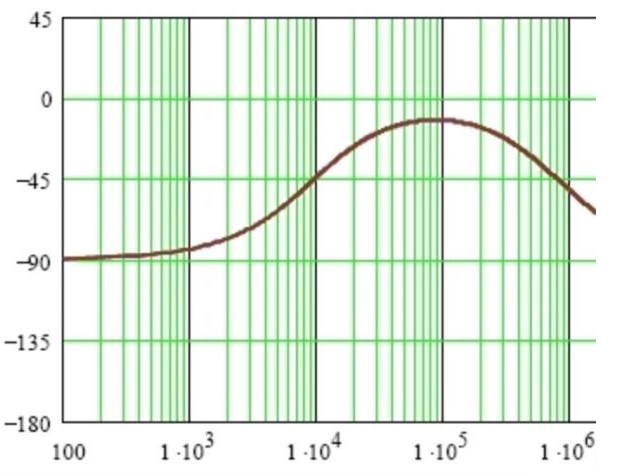由于放大电路中电抗元件的存在，放大电路对不同频率分量的信号放大能力是不相同的，而且不同频率分量的信号通过放大电路后还会产生不同的相移。因此，衡量放大电路放大能力的放大倍数也就成为频率的函数。
放大电路的电压放大倍数与频率的关系称为幅频特性,输出信号与输入信号的相位差与频率之间的关系称为相频特性。两者统称频率特性。


展开全文• 仿真分析了整个零序网络中各条出线的相频特性曲线,研究了接地方式对其相频特性的影响。分析表明:单相接地故障发生后,故障线路测量到的是所有健全线路组成的零序网络的相频特性,其特性取决于系统接地方式以及健全线路...
• 描述相频特性的一条曲线，通常称为相频特性曲线，指出响应的相位随着输入频率变化而变换的情况。通过频域分析给出相频特性与品质因数的关系及带通滤波电路的相频特性曲线与电路的品质因数的关系。
• 相频特性测试仪，用示波器显示数据. 用msp430作为处理器
• 已知y=x , x取值范围为〔0，2〕，如何用matlab 画幅频特性曲线和相频特性曲线matlab
• 基于STM32F407的幅频特性和相频特性测试仪，内含设计主要源码。
• 信号幅频相频特性的画法(频率响应法)。频率响应法和信号幅频相频特性的画法。信号与系统
• Φ(ω)是输出信号的相角与输入信号的相角之差，称为相频特性（相移角度随频率变化的特性叫相频特性） 在‘信号与系统’理论里边，有一个重要的概念，叫做“系统的频率响应函数”，它的物理意义是：当系统的输入是一...
1. 对于实数信号（要结合这份代码理解）：

A(ω)是输出信号的幅值与输入信号幅值之比，称为幅频特性。

Φ(ω)是输出信号的相角与输入信号的相角之差，称为相频特性（相移角度随频率变化的特性叫相频特性）

在‘信号与系统’理论里边，有一个重要的概念，叫做“系统的频率响应函数”，它的物理意义是：当系统的输入是一个幅值不变而频率变化的正弦波时，系统输出的幅值和相位随输入频率变化的关系，也就是系统的幅频特性和相频特性。

从数学的角度，系统的频率响应函数 H(jw) 等于系统输出y(t)的傅氏变换Y(jw)与输入x(t)的傅氏变换X(jw)的比值： H(jw) = Y(jw) / X(jw)

一般H(jw)是一个复数，它的模是‘幅频特性’；它的幅角就是‘相频特性’：这些特性在系统控制方面有重要的应用。

对于x = 3*cos(2*pi*f2*t+20/180*pi);的幅频特性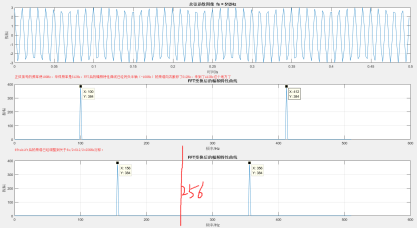对于x = 3*cos(2*pi*f1*t+thi1/180*pi) + 1*cos(2*pi*f2*t+thi2/180*pi);的幅频特性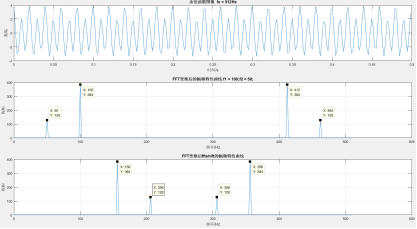对于x = 3*cos(2*pi*f1*t+thi1/180*pi) + 1*cos(2*pi*f2*t+thi2/180*pi);的相频特性与幅频特性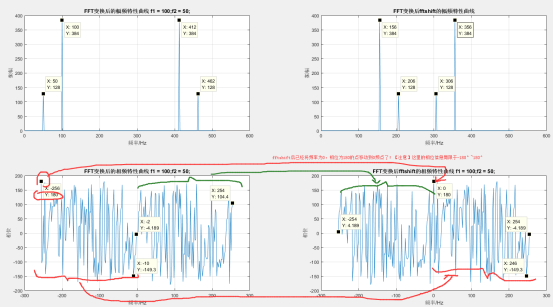Fftshift的作用：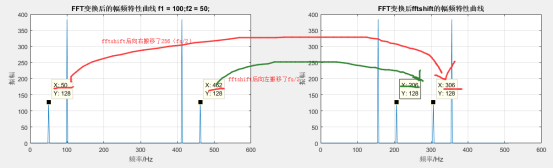结合图像来理解：http://blog.sina.com.cn/s/blog_5e1cdcaf0100yi41.html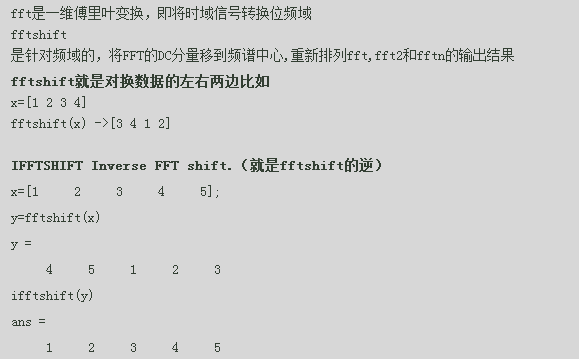2. 对于复数信号，且两个信号叠加的情况（要结合这份代码理解）：

%x=3*cos(2*pi*f1*t+thi1/180*pi)+1i*3*sin(2*pi*f1*t+thi1/180*pi)+1*cos(2*pi*f2*t+thi2/180*pi)+1i*1*sin(2*pi*f2*t+thi2/180*pi);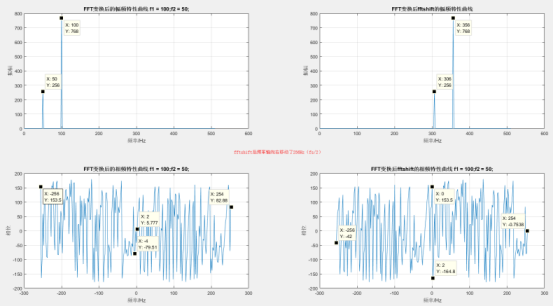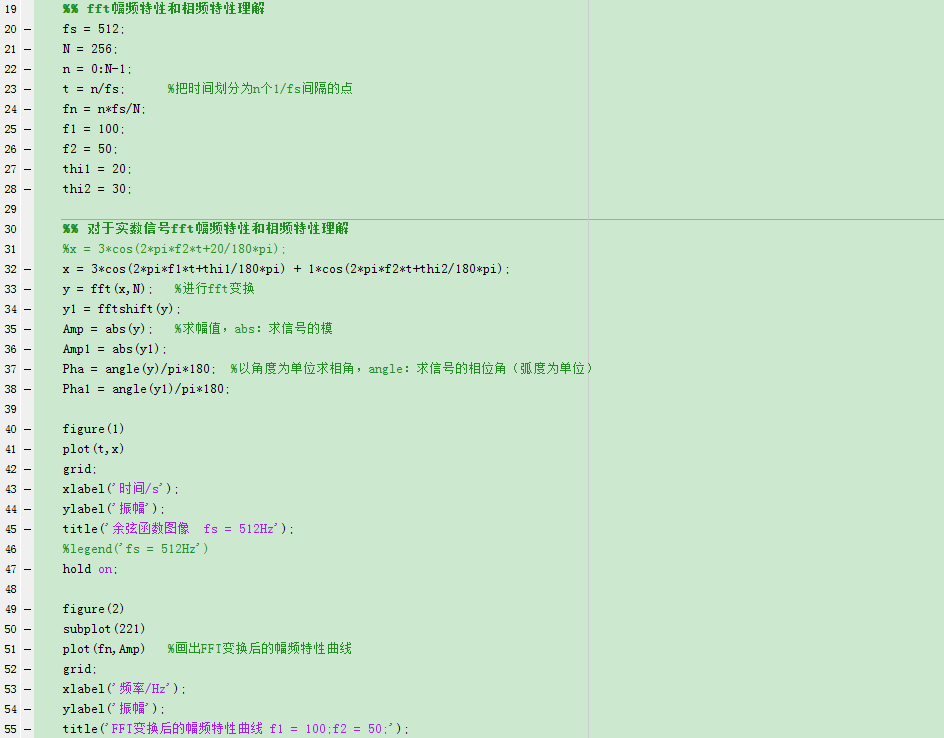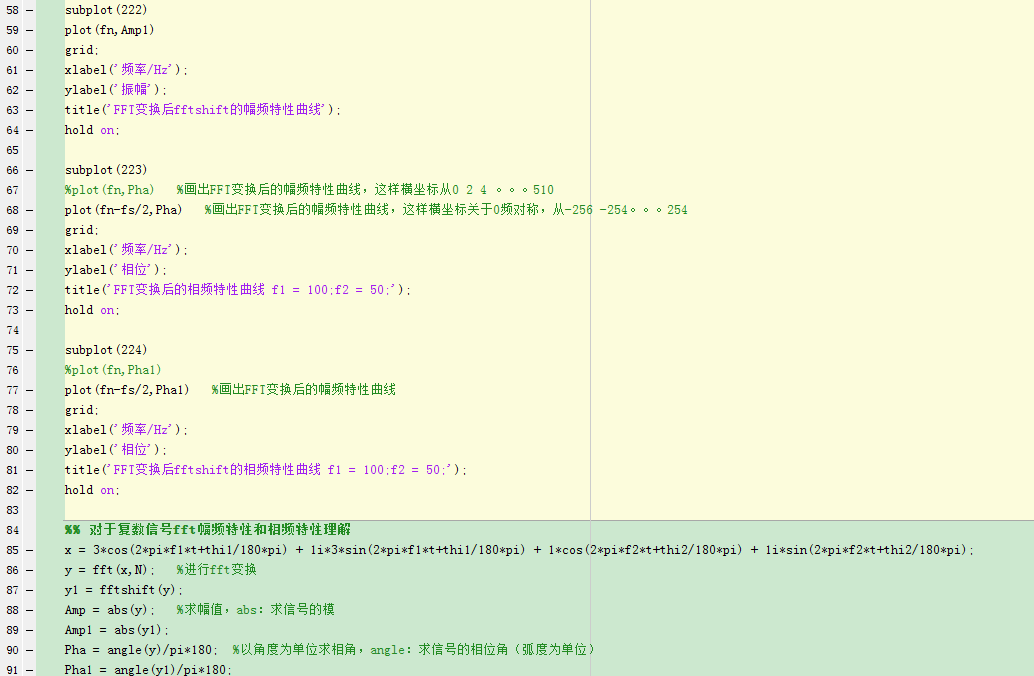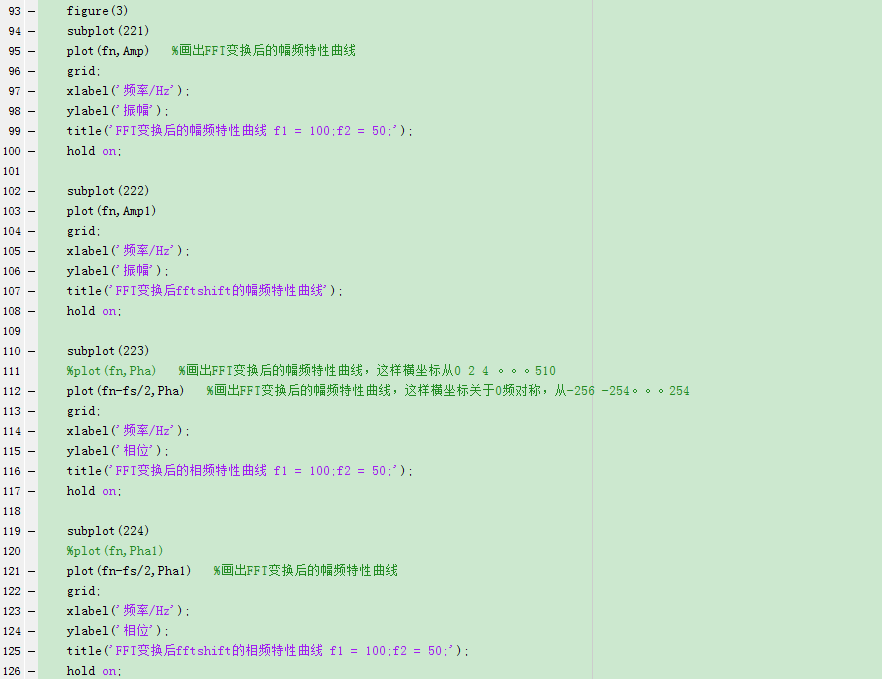相频响应的物理意义

研究一个线性时不变系统(LTI)，不仅可以用时域上的冲激响应h(n)来进行描述，也可以用频域上的频率响应H(w)来进行描述，而H(w)通常是一个复数，可分别用幅频响应和相频响应来表示。

幅频响应好理解，从物理概念上，幅频响应反映的是系统对不同频率信号的选择性。相频响应也有对应的非常明确的物理意义吗？回答是有的。从物理概念上，相频响应反映了系统对不同频率信号的处理时间。这点，我们也可以用一个例子来说明。

假定有甲乙丙三个同学，甲平时学习非常用功；乙平时除了学习，还喜欢关心很多其他的事情；丙则经常有点迷迷糊糊。三个人都报名参加了一个考试。到考试那天，甲乙都很早就起床，收拾妥当就往考点去了，丙则照常睡懒觉，等起来的时候都快到考试时间了，匆匆忙忙就往考点奔。这天考试的人很多，在进口处有保安查验证件，甲乙准备充分，很顺利地进入了考场，丙则因为太过匆忙，加上平时就经常犯迷糊，因为证件一时找不着了，连考场都没进得去。甲因为平时刻苦，很快就答完试卷出来了。乙则一直忙到考试结束。如果将考场当作一个系统，考生当作系统的输入。我们看到，甲乙两个信号都能通过系统，而丙则不能通过系统，这反映的是系统的选择性，即幅频响应。我们还看到，甲很快就出来了，乙则出来慢一些，这反映的是相频响应，即系统对不同信号的处理时间。

gy的：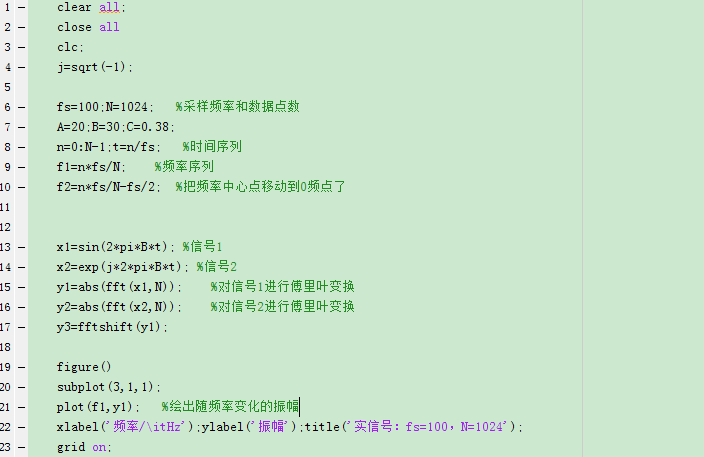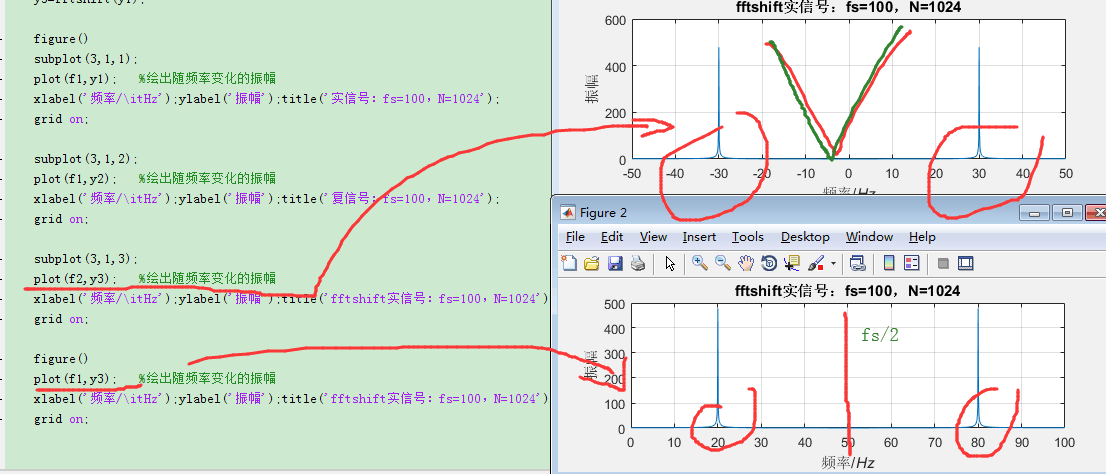上面第一幅图，实信号真实的频谱

展开全文• matlab仿真一阶低通滤波器幅频特性和相频特性.docx freqs模拟滤波器的频率响应语法：h=freqs(b,a,w)[h,w]=freqs(b,a)[h,w]=freqs(b,a,f)freqs(b,a)描述：freqs返回一个模拟滤波器的H(jw)的复频域响应(拉普拉斯格式)...matlab仿真一阶低通滤波器幅频特性和相频特性.docx freqs模拟滤波器的频率响应语法：h=freqs(b,a,w)[h,w]=freqs(b,a)[h,w]=freqs(b,a,f)freqs(b,a)描述：freqs返回一个模拟滤波器的H(jw)的复频域响应(拉普拉斯格式)请给出分子b和分母ah=freqs(b,a,w)根据系数向量计算返回模拟滤波器的复频域响应。freqs计算在复平面虚轴上的频率响应h，角频率w确定了输入的实向量，因此必须包含至少一个频率点。[h,w]=freqs(b,a)自动挑选200个频率点来计算频率响应h[h,w]=freqs(b,a,f)挑选f个频率点来计算频率响应h例子：找到并画出下面传递函数的频率响应Matlab代码：a=[10.41];b=[0.20.31];w=logspace(-1,1);logspace功能：生成从10的a次方到10的b次方之间按对数等分的n个元素的行向量。n如果省略，则默认值为50。freqs(b,a,w);Youcanalsocreatetheplotwith:h=freqs(b,a,w);mag=abs(h);phase=angle(h);subplot(2,1,1),loglog(w,mag)subplot(2,1,2),semilogx(w,phase)Toconverttohertz,decibels,anddegrees,use:f=w/(2*pi);mag=20*log10(mag);phase=phase*180/pi;算法：freqsuatesthepolynomialsateachfrequencypoint,thendividesthenumeratorresponsebythedenominatorresponse:s=i*w;h=polyval(b,s)./polyval(a,s)一阶低通滤波器频响计算：%**************************************************************************%一阶低通滤波器%取fH=1/(2Π*R*C)%s=jw=j*2Π*f%11%RC电路的电压增益AVH=———————=—————————(传递函数)%1+sRC1+j(f/fH)%11%电压增益的幅值(模)|AVH|=—————————=————————(幅频响应)%/——————/————————%\/1+(wCR)^2\/1+(f/fH)^2%VV%%电压增益的相位角ΦH=-arctg(wRC)=-arctg(f/fH)(相频响应)%**************************************************************************R=10000;%电阻的值C=0.0000001;%电容的值fZ=1;%H(e^jw)表达式分子的系数向量fM=[R*C,1];%H(e^jw)分母的系数向量%logspace功能：生成从10的a次方到10的b次方之间按对数等分的n个元素的行向量。n如果省略，则默认值为50。w=logspace(-1,5);%设定频率的变化范围%freqs根据系数向量计算返回一个模拟滤波器的H(e^jw)的复频域响应(拉普拉斯格式)g=freqs(fZ,fM,w);%求其频率响应%angle()返回复数元素的相角，单位为弧度x=angle(g);%求相频响应%abs()对复数返回其幅值y=abs(g);%求幅频响应subplot(2,1,1),%loglog()绘制双对数坐标，semilogx()/semilogy()绘制单对数坐标semilogx(w,x*180/pi)%画相频响应的曲线hw=line([0.1,1000],[-45,-45]);set(hw, LineWidth ,1);set(hw, color , r );xlabel( 角频率/(rad/s) );ylabel( 角度/° );gridon;subplot(2,1,2),%画幅频响应的曲线semilogx(w,20*log10(y))h=line([0.1,10000],[-20,-20]);set(h, LineWidth ,1);set(h, color , r );xlabel( 角频率/(rad/s) );ylabel( 幅值/dB );gridon;
展开全文• 基于相频特性与多频带分析的小电流接地系统故障选线rar,相频特性,多频带分析,小电流接地系统,单相接地故障选线,小波包,暂态零序电流
• 求H(s)=(928*s*(s^2+488*s+103100)*(s+418.6))/(s^2+169.4*s+14920)*(s^2+802.4*s+...jw带入的幅频特性|H(jw)|和相频特性arg(H(jw))代码咋写啊（还要画图） </p>matlab 有问必答
• 一级目录 二级目录 一.名词释义 1.频率特性 1.起因 : 由于放大电路中电抗元件的存在...3.相频特性 : 输出信号与输入信号的相位差与频率之间的关系称为相频特性. 2.波特图单位 dB : 增益 Deg : 角度单位 Rad : 弧度
一.名词释义

1.频率特性

1.起因 :  由于放大电路中电抗元件的存在,放大电路对不同频率分量的信号放大能力是不相同的(幅频特性提出点),而且不同频率分量的信号通过放大电路后还会产生不同的相移(相频特性提出点).因此衡量电路放大能力的放大倍数成为与频率相关的函数.
2.幅频特性 : 放大电路的电压放大倍数与频率的关系称为幅频特性.
3.相频特性 : 输出信号与输入信号的相位差与频率之间的关系称为相频特性.

2.波特图单位

dB : 增益
Deg : 角度单位

二.电路搭建三.波特图与频率特性详解
1.幅频特性

1.打开波特仪, 开始仿真, 按照如图所示调整图像, 将测量线放置到 5 Hz .2.计算 : 如图所示, 当信号频率约为 5 Hz时, 增益约为 -30dB.让我们来计算一下此时的放大倍数:

解 :
$\because$
放大倍数 : $\frac{u_o}{u_i}$
电压增益 : $K_u(dB)$
两者关系 : $K_u(dB)= 20lg\frac{u_o}{u_i}$
$\therefore$
放大倍数 : $K_1= \frac{u_o}{u_i}$
$=10^{\frac{K_u(dB)}{20}}$
$=10^{\frac{-30}{20}}$
$\approx 0.03162$

3.验证 : 粗浅地验证一下, 将两个测量线分别放置在输入输出两个波峰上(红 : 输入, 紫 : 输出),可以看到 :

$V_{inMAX} = 1.413V$
$V_{outMAX} = 44.913mV$
放大倍数 : $K_2= \frac{u_o}{u_i}$
$= \frac{V_{outMAX}}{V_{inMAX}}$
$=\frac{44.913mV}{1.413V}$
$\approx 0.03178$4.结论 :$K_1=0.03162 \approx K_2 =0.03178$ , 验证成功.

因为存在取余 ,放置测量线位置不精确等因素, 所以会造成一些误差.

2.相频特性

1.打开波特仪, 开始仿真, 按照如图所示调整图像, 将测量线放置到 5 Hz .2.已知 : 如图所示, 当信号频率约为 5 Hz时, 迟滞角度为
$D_1 =$ 88.186 Deg(Deg : °    –  角度)

3.验证 : 粗浅地验证一下, 将两个测量线分别放置在输入输出两个波峰上(红 : 输入, 紫 : 输出),可以看到 :
$\because$
输入与输出信号周期 : $T = \frac{1}{5}s = 200ms$
输出滞后输入信号时长 : $t = 49.926ms$
$\therefore$
迟滞角度 :
$D_2 = 49.926ms \times\frac{360°}{200ms} =89.8668$4.结论 : $D_1=88.186 \approx D_2=89.8668$

因为存在放置测量线位置不精确等因素, 所以会造成一些误差, 但不妨碍 验证成功

四.声明 : 个人见解,仅供参考


展开全文信号处理 经验分享
• 系统函数的幅频特性和相频特性分析参考与平台freqs(b,a,w)介绍例如输出 参考与平台 书籍《信号与系统》 matlab2016a freqs(b,a,w)介绍 对于有理分式，MATLAB 提供 freqs 函数处理方法。其调用格式为： H=freqs(b,a,w...
• 想必大家在学到信号与系统的时域和频域特性时，会对突然冒出的“群时延”、“相频特性为线性或非线性相位”所迷惑，我也在学习该知识点时特别懵逼很难理解为什么有了相位描述还来个群时延，后来在通信原理的学习中有...
• 截止频率为14Hz的一阶RC和二阶RC滤波器幅频相频特性及阶跃响应Matlab
• 土壤是典型的多孔介质，其阻抗特性直接决定接地系统的性能。 对各种土壤类型和含水量进行了土壤相频率特性测量。 本文发现，当电源频率（f）低时，土壤样品的阻抗角呈现出电容性。 随着频率增加，土壤阻抗角Swift...
• 目前我想标定加速度传感器的幅频特性曲线以及相位差 然后我用振动台标定加速度传感器，加速度传感器放在台面上，同时用激光位移计记录位移。输入为激光位移传感器的二次微分，输出为加速度传感器。 然后目前我想得到...matlab 有问必答 测试工具
• %取相位值对应相位角 clf figure(1) plot(w,20*log10(Hf)) %幅值变换为分贝单位 title('离散系统幅频特性曲线') figure(2) plot(w,Hx) title('离散系统相频特性曲线') 幅频特性曲线： 此时幅频特性曲线的横坐标为...
• 有关扫频仪设计的一些资料，包含22个文档的，看完基本上可以设计出来了。呵呵。。。。。。。
• 《matlab仿真一阶低通滤波器幅频特性和相频特性[计算机类]》由会员分享，可在线阅读，更多相关《matlab仿真一阶低通滤波器幅频特性和相频特性[计算机类](4页珍藏版)》请在人人文库网上搜索。1、freqs模拟滤波器的...
• 待处理原图 右击-properties-options，然后如图所示进行勾选 处理后bode图matlab
• 中科院上海光学精密机械研究所蔡海文课题组近日成功研发了一套低噪声单频激光器相频噪声特性测试仪器，并获得国家专利。该仪器既能保证测量完整性，同时又能保证测量稳定性，极具现实意义。本刊特邀请该课题组对这一...
• 不同截止频率的Bessel 滤波器幅频相频特性及阶跃响应特性分析Matlab
• 基于120°相差干涉和光场相位实时重构的窄线宽激光动态噪声特性测试方法,从时域频率调谐函数、频域频率噪声功率谱密度及其随时间的演化特征等方面,研究线性扫频激光器在不同扫频方式下动态相频噪声的细节特性。...
• RC电路 查看相频 幅频特性 使用.ac命令可以容易 清晰地看出相频 幅频特性： .ac命令的使用需要在激励源上加入一个小的ac信号（通常为1），然后进行.ac仿真即可, .ac仿真的命令可以参考博主的设置 构建正弦振荡 不...simulink cad
• 系统分DDS扫频信号源、被测网络、幅度和相位检测、控制模块及幅频、相频特性曲线显示等部分,在100Hz～100kHz范围内可自动步进测量被测网络的幅频特性和相频特性并自动设置频段范围，观察不同频段内网络的幅频特性和......